# Astronomy 12 - Spring 1999 (S.T. Myers)

## Solutions to Problem Set #4

Solutions:
1. If the stellar core mass available for fusion is a constant fraction of the total mass M, then the main sequence lifetime should be

tms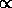M/L = 1010 ( M / Msun )/( L / Lsun) yr

from the given information. Then

 O8 : L = 1.7 x 105 Lsun M = 23 Msun ===> tms = 1.35 x 106 yr M8 : L = 1.2 x 10-3 Lsun M = 0.06 Msun ===> tms = 5 x 1011 yr

L = 4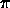R2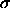T4    ===>    ( R / Rsun ) = ( L / Lsun )1/2 ( T / Tsun )-2

for Tsun = 5770 K, which gives

 O8 : T = 35000 K ===> R = 11.2 Rsun M8 : T = 2600 K ===> R = 0.17 Rsun

The central pressures are estimated by scaling the hydrostatic equilibrium equation dP/dr => P/R as usual

dP/dr = -GM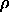/ r2    ===>    Pc/R = GM/ R2

or

Pc = GM/ R = 3GM2/(4R4)

which comes out to be

Pc = 2.7 x 1014 ( M / Msun )2 ( R / Rsun )-4 Pa.

The perfect gas law then gives the central temperature

Pc =kTc / (µmH)    ===>    Tc = (µmH/k)(GM/R)

or

Tc = 11.6 x 106 ( M / Msun ) ( R / Rsun )-1 K

assuming ionized hydrogen ((µ=0.5). Thus, we tabulate

 O8 : Pc = 9.1 x 1012 Pa Tc = 23.8 x 106 K M8 : Pc = 1.16 x 1015 Pa Tc = 4.1 x 106 K

Note that while the core temperatures are increasing as you move up the stellar sequence M -> G -> O (our Sun is G2), the core pressures are actually higher for lower mass stars! This explains why lower mass stars will have degenerate cores (and thus undergo helium flash) when the massive stars do not!

2. From pressure balance

P = n k T

we have for the outer (1) and inner (2) clouds

P/k = n1 T1 = n2 T2

and thus the core density is

n2 = (T1/T2) n1 = (200/10) 5 x 109 m-3 = 1011 m-3

which implies a mass density of= 2 mH n = 3.34 x 10-16 kg m-3

for H2. This gives a core radius of

R = [ 3M/(4) ]1/3 = 1.93 x 1015 m = 0.062 pc

The total thermal energy in the cloud core is

Uth = MkT / (2mH) = 4.13 x 1035 J

versus the gravitational potential energy

Ugr = GM2/R = 5.18 x 1046   > >   Uth

and thus this cloud core will collapse easily! We can write the collapse condition

GM2/R > MkT/(2mH)    ===>    M > RkT/(2mHG) = 1.2 x 1030 kg = 0.6 Msun

at constant radius. However, the problem asked for constant density which is different! Therefore, you should use core radius relation above to substitute in for R to get

M2/3 > [ 3/(4) ]1/3 [ kT / (2mHG) ]    ===>    M > [ 3/(4) ]1/2 [ kT / (2mHG) ]3/2

or

M > 4.1 x 1029 kg = 0.21 Msun

as our limiting mass.

3. If the radiation pressure and energy flux are related by

dFrad/dr = -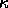Frad    ===>    dPrad/dr = - (/c) Frad

and then

Frad = L / (4r2)    ===>    dPrad/dr = - (L) / (4r2c).

dP/dr = - GM/ r2

which when equated to the radiative pressure gradient gives the Eddington limit

GM/ r2 = (L) / (4r2c)    ===>    LEd = 4Gc M /as expected. If the opacity= 0.04 m2 kg-1 =T / mH

from electron scattering as is expected in ultraluminous stars, then

LEd = 1.26 x 1031 ( M / Msun ) W = 3.28 x 104 ( M / Msun ) Lsun.

For 90 Msun, we find LEd = 2.95 x 106 Lsun! Note that with the usual scaling for high-mass stars

LM4

then we would expect 65.6 x 106 Lsun which is above the Eddington luminosity. Not surprisingly, 90 Msun is near the upper limit for main sequence stars.

4. The mass defect is

 4 1H =   4 x 1.007825 amu   = 4.031300 amu 4He = 4.002603 amu ___________________________________________ 0.028697 amu

or 4.765 x 10-29 kg. The mass fraction is

( 0.028697 / 4.031300 ) = 0.00712

as we got in lecture.

Using

E = m c2 = ( 4.765 x 10-29 kg) ( 3 x 108 m/s )2 = 4.29 x 10-12 J = 26.77 x 106 eV

for 1 eV = 1.602 x 10-19 J. Thus, each fusion gives off 26.77 MeV.

The solar luminosity Lsun = 3.83 x 1026 W, so

( 3.83 x 1026 J/s ) / ( 4.29 x 10-12 J/fusion) = 8.93 x 1037 fusion/s.

If the core mass is 0.13 Msun with X = 0.75 (mass fraction of hydrogen) then we have

MH,core = 0.75 x 0.13 = 0.0975 Msun = 1.95 x 1029 kg
of hydrogen in the core, and thus the fusion yield of the core is

Efus = 0.00712 MH,core c2 = 0.00712 (1.95 x 1029 kg) ( 9 x 1016 J/kg ) = 1.25 x 1044 J

of energy in the resevoir. Thus, the main-sequence lifetime is just

t = Efus/Lsun = ( 1.25 x 1044 J ) / ( 3.83 x 1026 J/s ) = 3.26 x 1017 s = 1.03 x 1010 yr

or 10.3 Gyr. You could have arrived at this number through various calculational routes.

For Helium burning

 3 4He =   3 x 4.002603 amu   = 12.007809 amu 12C = 12.000000 amu ___________________________________________ 0.007809 amu

The mass fraction is

( 0.007809 / 12.007809 ) = 6.50 x 10-4

so

1 kg 4He ==> 6.50 x 10-4 c2 = 5.84 x 1013 J

of energy.

As a red giant (technically on the horizontal branch), the Sun's core should essentially be all Helium, with a mass of

Mcore,He = 0.13 Msun = 2.6 x 1029 kg

which corresponds to

( 2.6 x 1029 kg ) x ( 5.84 x 1013 J/kg ) = 1.52 x 1043 J

total helium fusion energy available. At a luminosity of 100 Lsun, therefore, the Sun would have a lifetime of

( 1.52 x 1043 ) / ( 3.83 x 1028 J/s ) = 3.97 x 1014 s = 1.26 x 108 years

burning helium. All other things being equal (ie. the fraction of a particular type of star on any branch of the HR diagram merely reflecting the time it spends in any phase) then

t3a / tms = ( 1.26 x 108 yr ) / ( 1.03 x 1010 yr ) = 1.2 x 10-3

would be the fraction burning helium.

5. For a standard F0V star, Mv = +2.6 and B - V = +0.3, we see that we have a color excess of

CE = ( B - V)obs - ( B - V )true = 0.8 - 0.3 = 0.5

for our star. This implies a dust extinction of

Av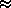3 CE = 1.5 mag

in the visual band (see notes p.52 or ZGS p.286). We therefore correct our distance modulus

mv - Mv = 5 log( d / 1pc ) - 5 + Av

obtaining

5 log( d / 1pc ) = 19.1 - 2.6 + 5 - 1.5 = 20

so d = 104 pc or 10 kpc.

smyers@nrao.edu   Steven T. Myers